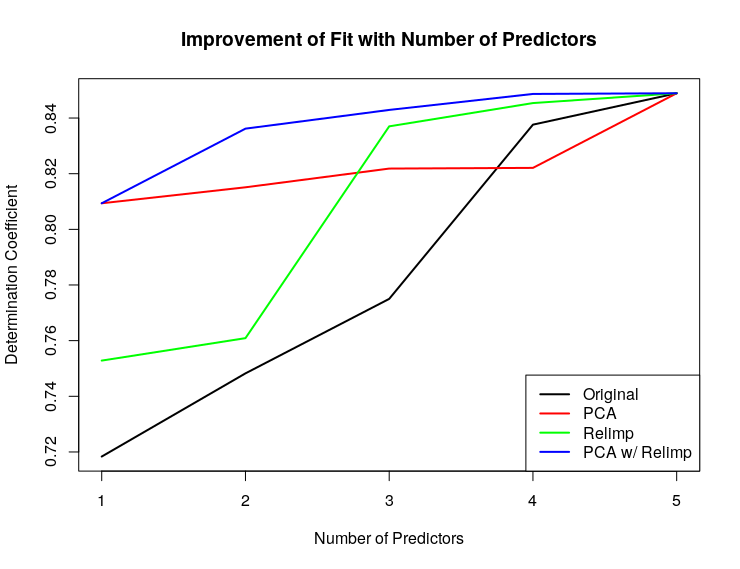# RelimpPCR - Relative Importance PCA Regression

## Acknowledgements

The concepts and ideas used to create this package/code were learned from Yuri Balasanov at the University of Chicago Master of Science in Analytics Program.

## Recent Additions

• Now able to handle matrices that, through PCA, produce very small eigenvalues, which would originally cause the calc.relimp() function to fail. RelimpPCR can now either a) iterative drop the last N PCA factors until a suitable matrix for calc.relimp() is produced, or b) drop the last M PCA factors, where M is a value set by the user.
• Now able to perform train/test split on data.
• Plots now show R2 progress for both train and test data sets.
• Added a prediction function, RelimpPCR.predict().

## Description

This package performs PCA dimensionality reduction in the context of a linear regression. In most cases, PCA dimensionality reduction is performed independent of the Y values for a regression. This captures the majority of the variance of the X values, but may not actually be the optimal dimensionality reduction solution for a regression against Y.

An alternative method, optimized for a regression against Y, is to use both PCA and a relative importance measure. This package applies PCA to a given data frame of X values, and then calculates the relative importance of each PCA factor against Y. It outputs ordered factors that are optimized for model fit. By performing dimensionality reduction with this method, an individual can achieve a the same r-squraed value as performing just PCA, but with fewer predictors.

## Installation

In a shell / command prompt build the package with “R CMD build RelimpPCR” (from the directory above “RelimpPCR”)
In a shell / command prompt install the package with “R CMD INSTALL RelimpPCR” (from the directory above “RelimpPCR”)

## Use

After importing the package, you can use the “RelimpPCR()” function to train a relative importance PCA regression.
After training a relative importnace PCA regression, you can use it for prediction with the “RelimpPCR.predict()” function.

## Example

Note in the below example that RelimpPCR achieves higher r-squared values faster than any other method.

Example of RelimpPCR on mtcars data